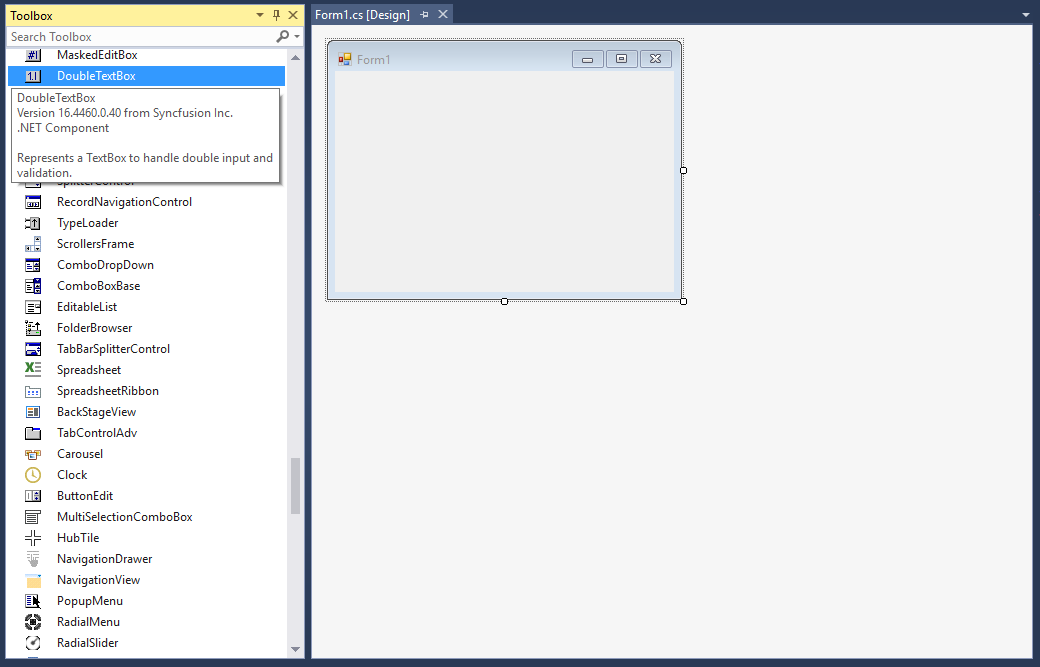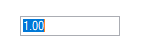# Getting Started with Windows Forms Double TextBox

11 Oct 20222 minutes to read

## Assembly deployment

Refer to the control dependencies section to get the list of assemblies or NuGet package that needs to be added as a reference to use the control in any application.

You can find more details about installing the NuGet packages in a Windows Forms application in the following link:

How to install nuget packages

### Create a simple application with DoubleTextBox

You can create a Windows Forms application with DoubleTextBox using the following steps:

### Create a project

Create a new Windows Forms project in Visual Studio to display the DoubleTextBox control.

The DoubleTextBox control can be added to an application by dragging it from the toolbox to a designer view. The Syncfusion.Shared.Base assembly reference will be added automatically:## Add control manually in code

1. Add the Syncfusion.Shared.Base assembly reference to the project:

2. Include the Syncfusion.Windows.Forms.Tools namespace.

``using Syncfusion.Windows.Forms.Tools;``
``Imports Syncfusion.Windows.Forms.Tools``
3. Create a DoubleTextBox instance, and add it to the window.

``````DoubleTextBox doubleTextBox1= new DoubleTextBox ();
``````Dim doubleTextBox1As DoubleTextBox = New DoubleTextBox ()## The maximum and minimum value constraints

You can set the maximum and minimum values using the MaxValue and MinValue properties of DoubleTextBox.

``````this.doubleTextBox1.MaxValue = 25;
this.doubleTextBox1.MinValue = 4;``````
``````Me.doubleTextBox1.MaxValue = 25
Me.doubleTextBox1.MinValue = 4``````

## Change number format

You can customize the number format using the NumberDecimalDigits, NumberDecimalSeparator, NumberGroupSeparator, NumberGroupSizes, and NumberNegativePattern properties of DoubleTextBox.

C# this.doubleTextBox1.DoubleValue = 24851343548781;
this.doubleTextBox1.NumberDecimalDigits = 3;
this.doubleTextBox1.NumberDecimalSeparator = “-“;
this.doubleTextBox1.NumberGroupSeparator = “;”;
this.doubleTextBox1.NumberGroupSizes = new int[] { 4 };
this.doubleTextBox1.NumberNegativePattern = 2;
VB Me.doubleTextBox1.DoubleValue = 24851343548781
Me.doubleTextBox1.NumberDecimalDigits = 3
Me.doubleTextBox1.NumberDecimalSeparator = “-“
Me.doubleTextBox1.NumberGroupSeparator = “;”
Me.doubleTextBox1.CurrencyGroupSizes = New Integer() {3}
Me.doubleTextBox1.NumberNegativePattern = 2